# How to use a point-and-click method to create a formula in Google Sheets?

Last updated Jul 09, 2022

Using the point-and-click method, you can now use the mouse pointer to add cell references to a formula by simply clicking on the desired cells. Learn how to create formulas fast and easily using this method.

The point and click method are usually preferred for adding cell references to formulas and functions, as it reduces errors that can occur when misreading or typing incorrectly. It also saves time and effort when formulas are created since most people see the data rather than the reference to the cell. Let’s get started on how to use this method.

How to create a formula using a point-and-click method

Step 1: Select the cell to write the formula and type the equals (=) sign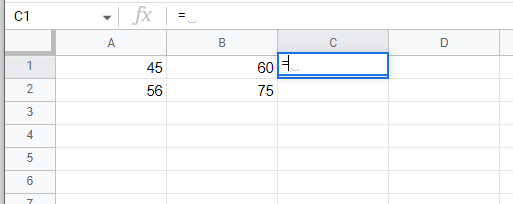Step 2: Choose the first cell to add to the formula. The cell reference appears in the formula, and a dashed line surrounds it.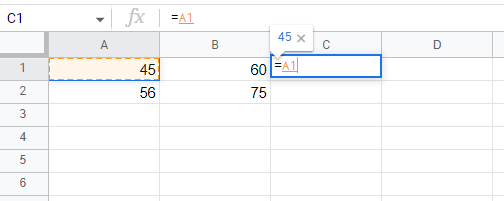Step 3: Enter the operator into the formula after the first cell reference by pressing the mathematical operator key on the keyboard (such as plus or minus).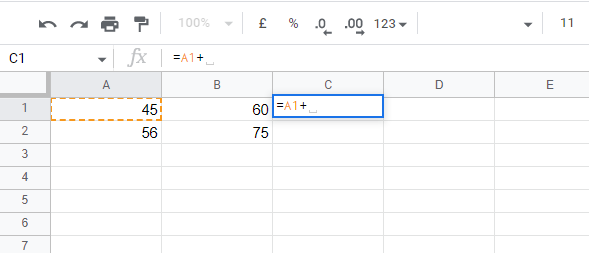Step 4: Add the second cell to the formula. A dashed red line appears around the second cell referenced in the formula.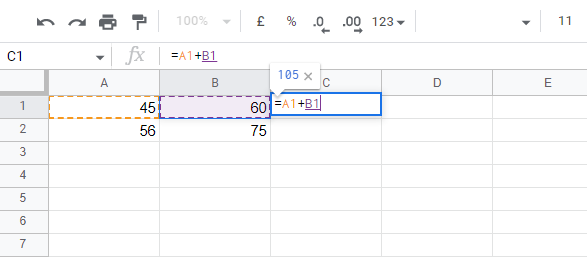Step 5: Add operators and cell references until the formula is complete.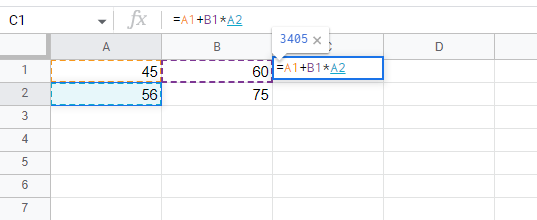Step 6: Once the formula has been completed, press Enter to see the answer displayed in the cell.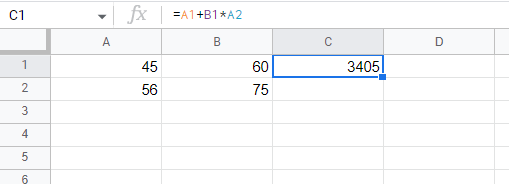Conclusion

To conclude, we have learned how to create formulas using the point-and-click method. The main advantage of this method is that there is less chance of errors

 Article Contributed By :106 Views
Projects By category
Categories